# 显示区域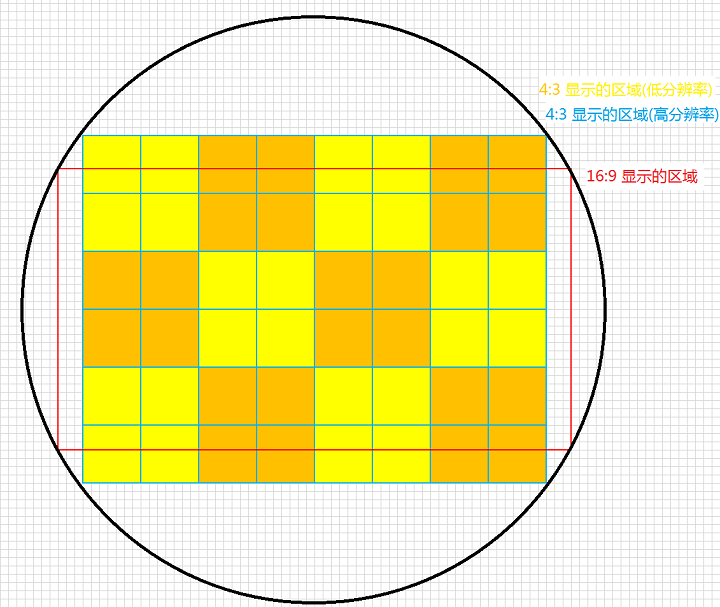# 预览分辨率

1、全屏预览

2、预览尽可能没有拉伸

3、预览尽可能清晰

## 全屏预览

### 隐藏状态栏、标题栏、虚拟按键栏

1、在 activity 中加入代码

### 获取屏幕分辨率

1、HardwareInfoUtil 的方法

2、调用

# 拍照分辨率

## 需求

1、裁剪图片在预览区域中指定方框中的区域，例如：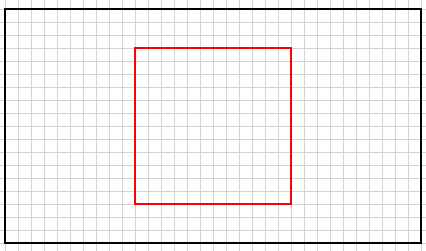2、裁剪区域的图片分辨率为 1080x1080

3、裁剪区域的图片尽量不失真

## 裁剪图片在预览区域中指定方框中的区域

### 裁剪图片在预览区域中指定方框中的区域

1、BitmapUtil 的方法

2、调用

#### 预览分辨率和拍照分辨率不一致

1、获取预览分辨率，比如 previewRatio（在上面设置预览分辨率时保存，且值为宽:长，因为容易整除），拍照分辨率 pictureRatio

2、获取因拍照分辨率和预览分辨率不一致导致的自动被裁剪/添加的区域，比如预览分辨率是 16:9，拍照分辨率是 4:3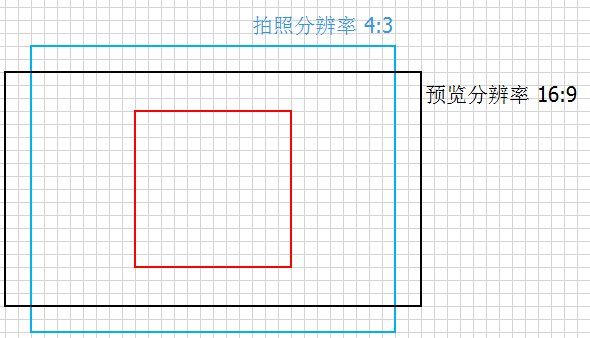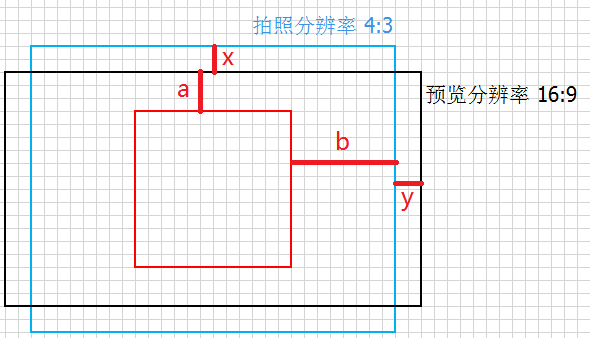3、x 计算

$$lx’ = \frac{16a * pictureRatio}{2}$$
，如果不是因为相机传感器是圆形的，实际 x 应该是
$$x’ = \frac{16a * pictureRatio - 16a * previewRatio}{2 * 16a * pictureRatio} = \frac{pictureRatio - previewRatio}{2 * pictureRatio}$$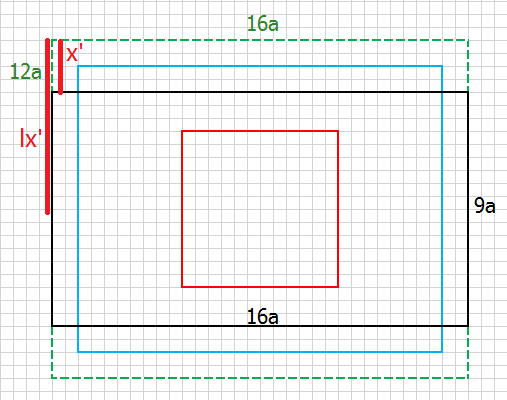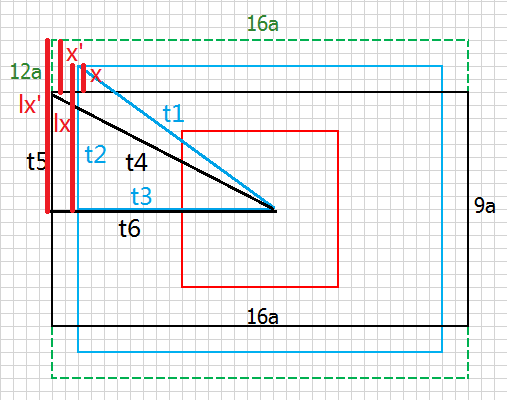$$t1 = t4$$

$$t2^2 + t3^2 = t5^2 + t6^2$$

$$t2 = t3 * pictureRatio，t5 = t6 * previewRatio$$

$${(t3 * pictureRatio)}^2 + t3^2 = {(t6 * previewRatio)}^2 + t6^2$$

$${(1 + pictureRatio)}^2 * t3^2 = {(1 + previewRatio)}^2 * t6^2$$

$$\frac{t3}{t6} = \sqrt{\frac{(1 + previewRatio)}{(1 + pictureRatio)}}$$

$$\frac{lx}{lx’} = \frac{t3}{t6} = \sqrt{\frac{(1 + previewRatio)}{(1 + pictureRatio)}}$$

$$x = x’ - \frac{lx’ - lx}{lx’} = x’ - 1 + \frac{lx}{lx’} = \frac{pictureRatio - previewRatio}{2 * pictureRatio} - 1 + \sqrt{\frac{(1 + previewRatio)}{(1 + pictureRatio)}}$$

4、y 计算

$$y = \frac{t6 - t3}{t3} = \frac{t6}{t3} - 1 = \frac{1}{\sqrt{\frac{(1 + previewRatio)}{(1 + pictureRatio)}}} - 1$$

5、BitmapUtil 的方法

6、调用

## 裁剪区域的图片尽量不失真

---------------------本文结束---------------------